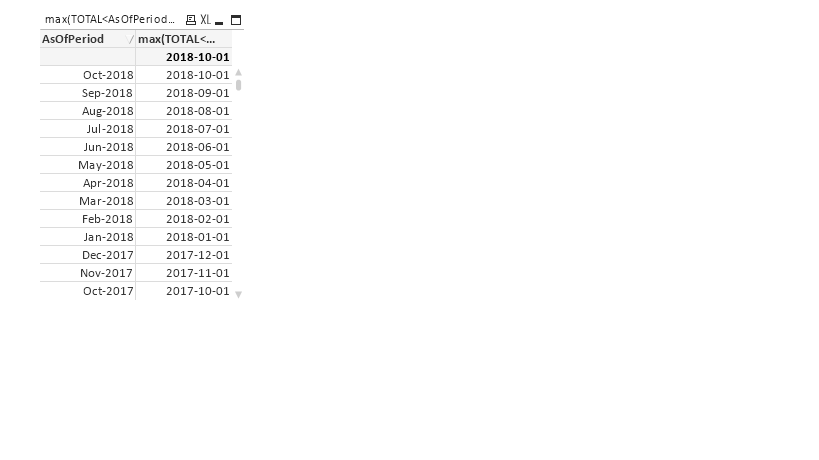# QlikView App Development

Discussion Board for collaboration related to QlikView App Development.

Announcements
Coming your way, the Qlik Data Revolution Virtual Summit. October 27-29. REGISTER
cancel
Showing results for
Did you mean:
HighlightedContributor II

## Max month inside the P() as per the dimension gives always the same value

Below is the expression and a table where for Each AsOfPeriod the max submission date is different, but when I'm putting the below expression for every AsOfPeriod it's taking the oct submission date under the P().  Does P() Doesn't allow to calculate the max date as per the Dimension.

SUM(AGGR(If(
Count(DISTINCT {<AsOfPeriod=,PeriodType={'Current month'}>}%ProdLocaleKey)>0,
if((sum({<%ProdLocaleKey=p({\$<AsOfPeriod=,Retailer=,[Submission date]={"\$(=max([Submission date]))"}>}%ProdLocaleKey)>}RatingSum) /
sum({<%ProdLocaleKey=p({\$<AsOfPeriod=,Retailer=,[Submission date]={"\$(=max([Submission date]))"}>}%ProdLocaleKey)>}Reviews))>=4.25,1,0)),AsOfPeriod,ProductID,Market))2 Replies
HighlightedMVP

Use case of P() and E() are the same where set analysis allows. Perhaps this?

SUM({<AsOfPeriod=,Retailer=,[Submission date]={"\$(=max([Submission date]))"}>} AGGR(If(
Count(DISTINCT {<AsOfPeriod=,PeriodType={'Current month'}>}%ProdLocaleKey)>0,
if((sum({<%ProdLocaleKey=p({\$<AsOfPeriod=,Retailer=,[Submission date]={"\$(=max([Submission date]))"}>}%ProdLocaleKey)>}RatingSum) /
sum({<%ProdLocaleKey=p({\$<AsOfPeriod=,Retailer=,[Submission date]={"\$(=max([Submission date]))"}>}%ProdLocaleKey)>}Reviews))>=4.25,1,0)),AsOfPeriod,ProductID,Market))

Before develop something, think If placed (The Right information | To the right people | At the Right time | In the Right place | With the Right context)
HighlightedContributor II

Thing the max submission date is not changing as per the dimension , is there a way that it gives me max month for every as of period in the dimension , row by row?